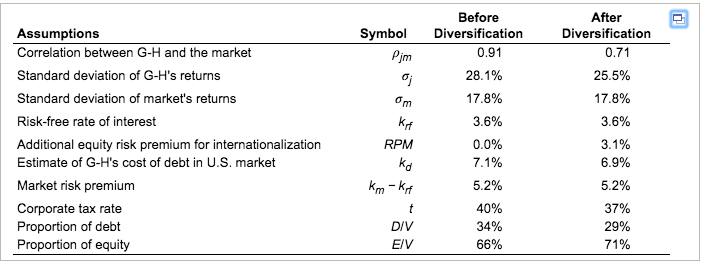# Solved Question: Genedak-Hogan’s WACC and Effective Tax Rate

Get premium Solution to all your Homework Assignments exclusively on writerkingdom. We have CFA/MBA writers to provide customized model answers to your every academic problem

## Description

Question:

Genedak-Hogan’s WACC and Effective Tax Rate. Use the table in the popup window, to answer the problem.Genedak-Hogan is an American conglomerate that is actively debating the impacts of international diversification of its operations on its capital structure and cost of capital. The firm is planning on reducing consolidated debt after diversification. Senior management at Genedak-Hogan are actively debating the implications of diversification on its cost of equity. All agree that the company’s returns will be less correlated with the reference market return in the future, the financial advisors believe that the market will assess an additional 3.1% risk premium for “going international” to the basic CAPM cost of equity. Many MNEs have greater ability to control and reduce their effective tax rates when expanding international operations. Assume that Genedak-Hogan was able to reduce its consolidated effective tax rate from 40% to 37% after international diversification.

1. Without the hypothetical additional risk premium, what is Genedak-Hogan’s cost of equity before international diversification of its operations? ​(Round to two decimal​ places.)

2. Calculate the weighted average cost of capital for Genedak-Hogan before and after international diversification. ​(Round to two decimal​ places.)

3. Adding the hypothetical risk premium to the cost of equity (an added 3.1% to the cost of equity because of international diversification), what is the firm’s WACC before and after international diversification? ​(Round to two decimal​ places.)

4. If Genedak-Hogan was able to reduce its consolidated effective tax rate from 40% to 37%  , what would be the impact on its WACC?

Part (1):-

We know that Correlation (X, Y) = Covariance (X, Y) / Standard Deviation of X *Standard Deviation Y

Here,

Before internationalization

0.91= Covariance (X, Y) /(0.281*0.178)

Covariance = 0.04551638

Similarly after internationalization

Covariance= 0.0322269

Now, calculating beta.,

Beta = Covariance(X, Y) /Variance of market return

This gives beta before internationalization= 1.43657

and after internationalization= 1.0171348

CAPM= Risk free rate of return + risk premium * beta

Before internationalization

Cost of equity (capm) = 0.036+1.43657*0.052=11.07%

Part2:-

WACC= proportion of equity* cost of equity+ proportion of debt * cost of debt

Before internationalization

WACC= 0.66*0.1107+.34*0.071=9.72%

After internationalization

WACC=0.71*0.1107+0.29*0.069= 9.86%

Part 3:-new beta was calculated in part 1

Cost of equity= 0.036+.1.0171348*(0.052+0.031) =12.04%

Before internationalization

WACC=0.1204*.66+0.071*0.34= 10.36%

After internationalization

WACC=0.1204*0.71+0.069*0.29= 10.549%

Part 4:-

WACC= Proportion of equity * cost of equity + proportion of debt* cost of debt (1-tax rate)

Before internationalization

WACC= 0.66*0.1204+0.34*0.071(1-0.40)=9.394%

After internationalization

WACC=0.71*0.1204+0.29*0.069(1-0.37)=9.809%

## Reviews

There are no reviews yet.